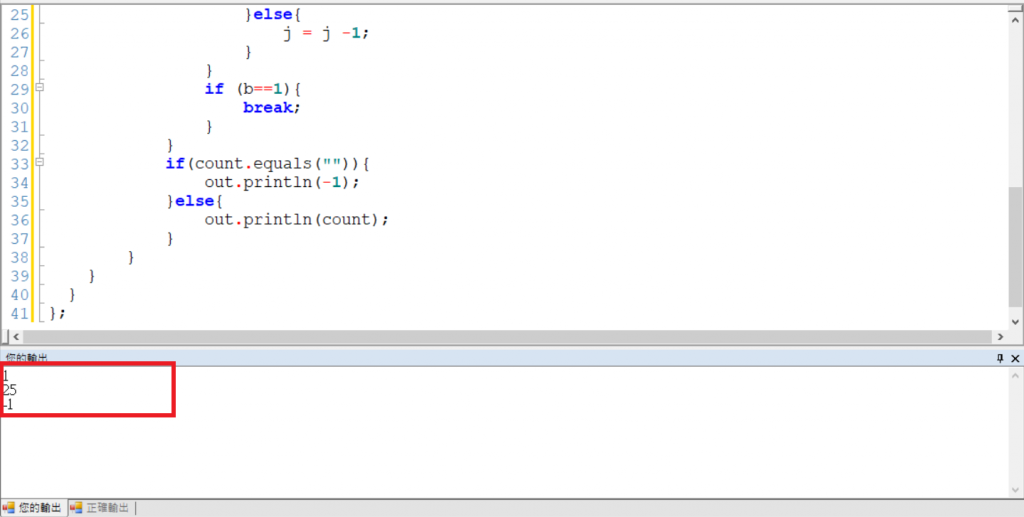#DAY 25
0

## [iT鐵人賽Day25]練習題(4)

3
1
10
123456789

``````import java.util.*;
import static java.lang.System.*;
public class main{
public static void main(String[] args) {
Scanner sc=new Scanner(System.in);
int a=sc.nextInt();
for (int i=0;i<a;i++){
int b=sc.nextInt();
String count = "";
if(b==1 || b==0){
out.println(b);
}else{
int j = 9;
while(true){
if (b%j==0){
count = String.valueOf(j)+ count;
b = b/j;
}else{
if(j==2){
count = "";
break;
}else{
j = j -1;
}
}
if (b==1){
break;
}
}
if(count.equals("")){
out.println(-1);
}else{
out.println(count);
}
}
}
}
};
``````

int a=sc.nextInt()的意思是a有3筆資料
int b=sc.nextInt()就是b會等於1,10,123456789

if(count.equals("")){
out.println(-1);